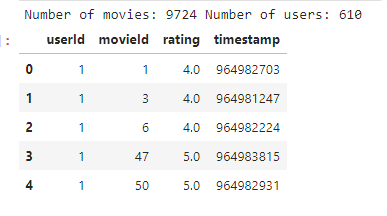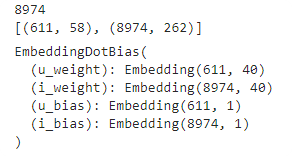# Collaborative filtering - different size of Embedding matrix

Hi,

I’ve tried collaborative filtering, by below example using files from MovieLens-Latest.

``````from fastai.collab import *
from fastai.tabular import *

#'http://files.grouplens.org/datasets/movielens/ml-latest-small.zip'
#path = untar_data(URLs.ML_SAMPLE)
#print(path.ls())

all_movies = ratings['movieId'].unique().astype(str)
all_users = ratings['userId'].unique().astype(str)

print('Number of movies:', len(all_movies),'Number of users:', len(all_users))
``````I hope to get the embedding size for items: 9724, and for user 610, but when I divide data and split what I’ve got is only: 8974:

``````user,title,rating = 'userId','movieId','rating'
data = CollabList.from_df(ratings, cat_names=[user,title],procs=Categorify)
data_split = data.split_by_rand_pct(valid_pct=0.2, seed=200).label_from_df(cols=rating)
print('classes: ', len(data_split.x.classes['movieId']))
``````
``````data_bunch = data_split.databunch()
y_range = [0,5.5]
learn = collab_learner(data_bunch, n_factors=40, y_range=y_range, wd=1e-1)

print(len(learn.data.x.classes['movieId']))
print(learn.data.get_emb_szs())
learn.model
``````and a model size is not that I expected. I notice that if I use `valid_pct=0` than I get the correct size. I thought that size for weights and biases should be the same as the size of the classes. What could cause the different size of the weights ?

This is why you see the right number when setting a validation pct to 0. Another way to solve this would be to carefully compute validation indices so that all the classes are present in the training set and not use a random split. You would then use `split_by_idx` instead of `rand_split_by_pct`.